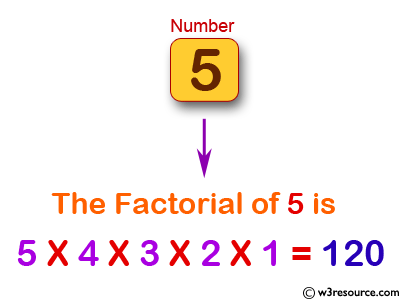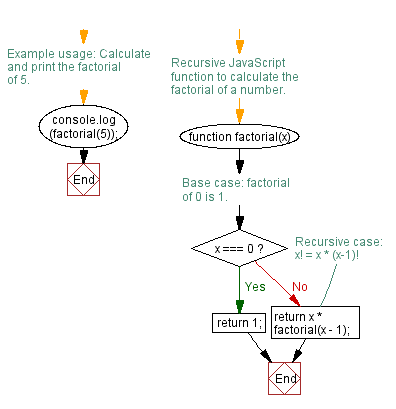# JavaScript: Calculate the factorial of a number

## JavaScript Function: Exercise-1 with Solution

Write a JavaScript program to calculate the factorial of a number.

In mathematics, the factorial of a non-negative integer n, denoted by n!, is the product of all positive integers less than or equal to n. For example, 5! = 5 x 4 x 3 x 2 x 1 = 120

Pictorial Presentation:Sample Solution:-

HTML Code:

``````<!DOCTYPE html>
<html>
<meta charset=utf-8 />
<title>Calculate the factorial of a number.</title>
<body>
</body>
</html>
```
```

JavaScript Code:

``````function factorial(x)
{

if (x === 0)
{
return 1;
}
return x * factorial(x-1);

}
console.log(factorial(5));
```
```

Output:

```120
```

Flowchart:Live Demo:

See the Pen javascript-recursion-function-exercise-1 by w3resource (@w3resource) on CodePen.

Improve this sample solution and post your code through Disqus

What is the difficulty level of this exercise?

Test your Programming skills with w3resource's quiz.

﻿

## JavaScript: Tips of the Day

Creates an object from the given key-value pairs

Example:

```const tips_objectFromPairs = arr => arr.reduce((a, [key, val]) => ((a[key] = val), a), {});
console.log(tips_objectFromPairs([['x', 2], ['y', 4]]));
```

Output:

```[object Object] {
x: 2,
y: 4
}
```